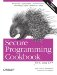# Representing a Dynamically Sized Numerical Vector

Problem

You want a type for manipulating numerical vectors with dynamic size.

Solution

You can use the valarray template from the header. Example 11-15 shows how you can use the valarray template.

Example 11-15. Using valarray

```#include
#include

using namespace std;

int main( ) {
valarray v(3);
v = 1; v = 2; v = 3;
cout << v << ", " << v << ", " << v << endl;
v = v + v;
cout << v << ", " << v << ", " << v << endl;
v /= 2;
cout << v << ", " << v << ", " << v << endl;
}```

The program in Example 11-15 will output the following:

```1, 2, 3
2, 4, 6
1, 2, 3```

Discussion

Despite its name, vector is not intended to be used as a numerical vector; rather, the valarray template is. The valarray is designed so that C++ implementations, especially those on high-performance machines, can apply specialized vector optimizations to it. The other big advantage of valarray is that it provides numerous overloaded operators specifically for working with numerical vectors. These operators provide such functionality as vector addition and scalar multiplication.

The valarray template can also be used with the standard algorithms like a C-style array. See Example 11-16 to see how you can create iterators to the beginning of, and one past the end of, a valarray.

Example 11-16. Getting iterators to valarray

```template
T* valarray_begin(valarray& x) {
return &x;
}

template
T* valarray_end(valarray& x) {
return valarray_begin(x) + x.size( );
}```

Even though it appears somewhat academic, you should not try to create an end iterator for a valarray by writing &x[x.size( )]. If this works, it is only by accident since indexing a valarray past the last valid index results in undefined behaviour.

The lack of begin and end member functions in valarray is decidedly non-STL-like. This lack emphasizes that valarray does not model an STL container concept. Despite that, you can use valarray with any of the generic algorithms where a random-access iterator is required.Secure Programming Cookbook for C and C++: Recipes for Cryptography, Authentication, Input Validation & More
ISBN: 0596003943
EAN: 2147483647
Year: 2006
Pages: 241